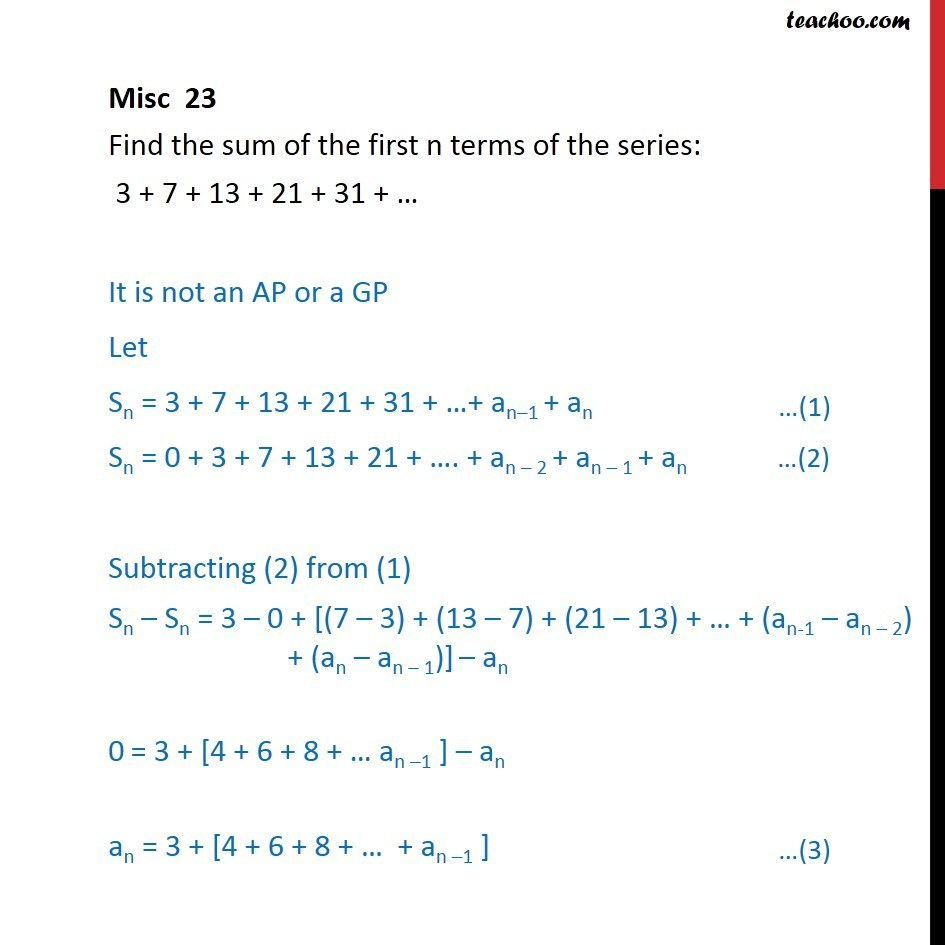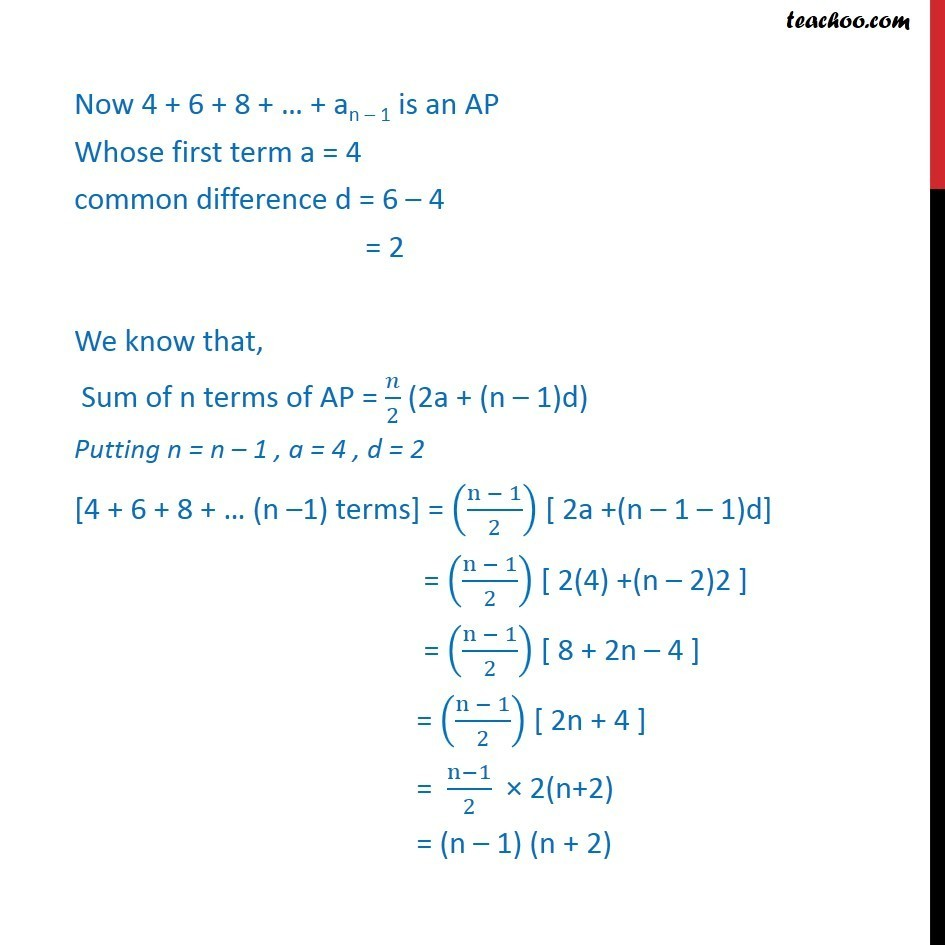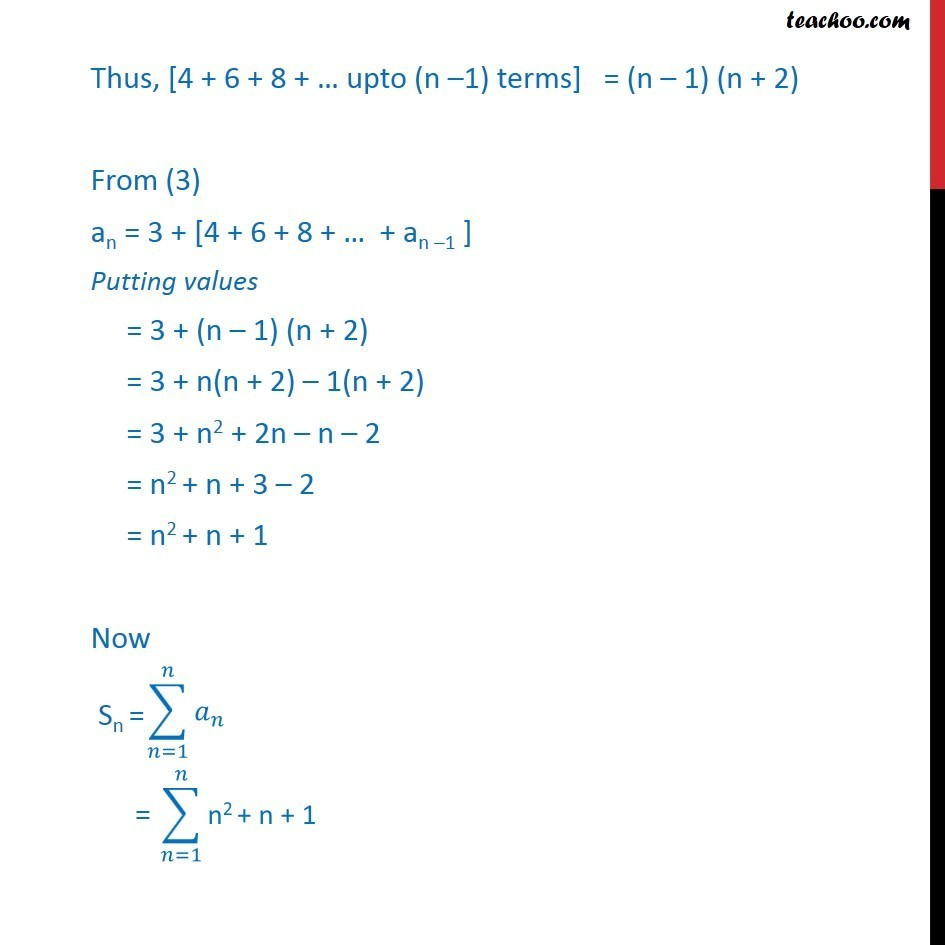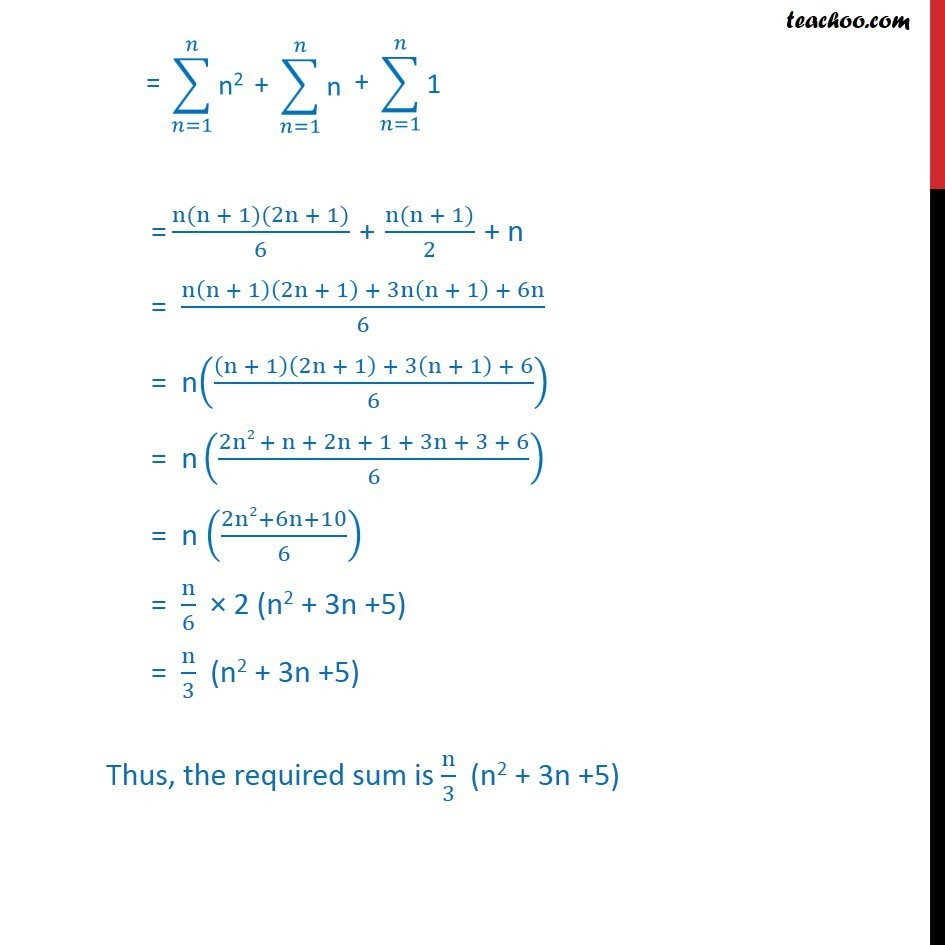Miscellaneous

Chapter 8 Class 11 Sequences and Series
Serial order wiseLearn in your speed, with individual attention - Teachoo Maths 1-on-1 Class

### Transcript

Misc 23 Find the sum of the first n terms of the series: 3 + 7 + 13 + 21 + 31 + … It is not an AP or a GP Let Sn = 3 + 7 + 13 + 21 + 31 + …+ an–1 + an Sn = 0 + 3 + 7 + 13 + 21 + …. + an – 2 + an – 1 + an Subtracting (2) from (1) Sn – Sn = 3 – 0 + [(7 – 3) + (13 – 7) + (21 – 13) + … + (an-1 – an – 2) + (an – an – 1)] – an 0 = 3 + [4 + 6 + 8 + … an –1 ] – an an = 3 + [4 + 6 + 8 + … + an –1 ] Now 4 + 6 + 8 + … + an – 1 is an AP Whose first term a = 4 common difference d = 6 – 4 = 2 We know that, Sum of n terms of AP = 𝑛/2 (2a + (n – 1)d) Putting n = n – 1 , a = 4 , d = 2 [4 + 6 + 8 + … (n –1) terms] = ((n − 1)/2) [ 2a +(n – 1 – 1)d] = ((n − 1)/2) [ 2(4) +(n – 2)2 ] = ((n − 1)/2) [ 8 + 2n – 4 ] = ((n − 1)/2) [ 2n + 4 ] = (n−1)/2 × 2(n+2) = (n – 1) (n + 2) Thus, [4 + 6 + 8 + … upto (n –1) terms] = (n – 1) (n + 2) From (3) an = 3 + [4 + 6 + 8 + … + an –1 ] Putting values = 3 + (n – 1) (n + 2) = 3 + n(n + 2) – 1(n + 2) = 3 + n2 + 2n – n – 2 = n2 + n + 3 – 2 = n2 + n + 1 Now = (n(n + 1)(2n + 1))/6 + (n(n + 1))/2 + n = (n(n + 1)(2n + 1) + 3n(n + 1) + 6n)/6 = n(((n + 1)(2n + 1) + 3(n + 1) + 6)/6) = n ((2n2 + n + 2n + 1 + 3n + 3 + 6)/6) = n ((2n2+6n+10)/6) = n/6 × 2 (n2 + 3n +5) = n/3 (n2 + 3n +5) Thus, the required sum is n/3 (n2 + 3n +5)